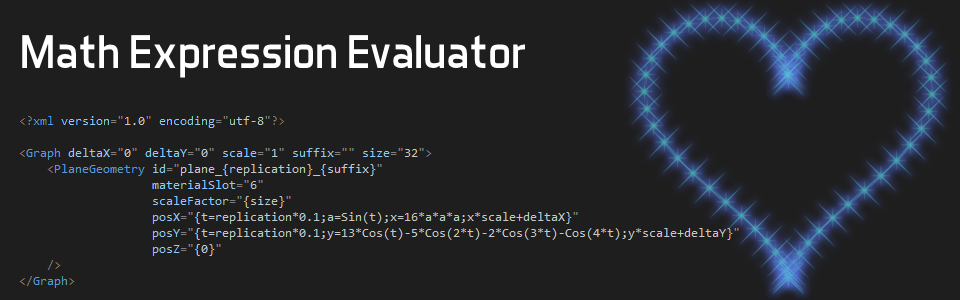## Mathematical Expression Evaluator

Posted on January 14, 2015Our latest addition to the Murl Engine is a practical mathematical expression evaluator. The evaluator allows you to specify a mathematical expression as parameter value in resource XML files. (updated 24.05.2015)

The ability to use mathematical expressions instead of fixed values is often very beneficial. You can set up graph objects directly within XML graph resource files without the need of additional C++ code. This is especially useful when you are working with instance replications. For instance, the feature image shows an example where a mathematical expression is used to align some `PlaneGeometry` objects along a heart shaped path.

To specify a mathematical expression, enclose the expression in curly braces. This is the same notation as with passed attribute values. Here is a simple example:

`<PlaneGeometry id="plane_{replication}" posX="{replication*10}" posY="0"/>`

Combining Expressions

A sequence of expressions can be defined by using a semicolon separated list. The last expression is assigned to the attribute, e.g.:

`	<PlaneGeometry posX="{R=400;r=100;b=65;t=replication*0.01;(R-r)*Sin(t)-b*Sin((R-r)/r*t)}" />`

It is also possible to nest expressions which will result in a separate evaluation for each nested expression:

`	<PlaneGeometry posX="{{2*3}+6}}" />`

Datatypes

The evaluator supports the following data types:

Double, SInt32, Bool, String

The correct data type is determined automatically. An explicit type conversion can be done in functional notation:

`	<PlaneGeometry posX="{SInt32(replication*0.1)}" />`

To specify a `String`, enclose the characters in single quotes:

`	<Node id="{'ab'+String('c')+BoolToString(1)}" />`

The result for the expression above would be:

abctrue

Conditional statements

The `if` function can be used for conditional if/else expressions.

`	<PlaneGeometry posX="{if(replication > 10, 100, 0)}" />`

A C++ equivalent for this expression would be:

```	if (replication > 10)
{
posX = 100;
}
else
{
posX = 0;
}```

Alternatively the `switch` function can be used to create a conditional expression. The `switch` function expects a condition parameter followed by a number of case parameters. The condition parameter is clamped to the range [0, number_of_case_parameters-1]. Example:

`	<PlaneGeometry posX="{switch(replication * 0.1, 100, 200, 50)}" />`

In this example the condition parameter is clamped to the range [0,2]. Therefore 100 is assigned to instances 0 to 9, 200 to instances 10 to 19 and 50 to all others.

Equivalent C++ code for the expression above would be:

```	SInt32 condition = SInt32(replication * 0.1)
if (condition < 0)
{
condition = 0;
}
switch(condition)
{
case 0: posX = 100; break;
case 1: posX = 200; break;
default: posX = 50;
}
```

Random Number Generator

A global Random Number Generator (RNG) is provided to allow the usage of random numbers. The RNG is always initialised with the same seed value and therefore is always generating the same sequence of numbers. The function `Seed(value)` can be used to set a new seed value. The function `Seed()` without parameters uses the current system time as seed value.

`	<Instance graphResourceId="graph_plane" replications="{Seed(42);10}"/>`

For example, `RandSInt(start, end)` can be used to draw a new random number.

`	<PlaneGeometry posX="{replication*10}" posY="{RandSInt(-100,100)}" />`

C++ Default Parameters

You can define global default parameters in the `IApp::Configure()` method and read those parameters in your mathematical expression. The method `GetDefaultGraphParameters()` has been added to the `IEngineConfiguration` class for that purpose.

```	Bool App::MyApp::Configure(IEngineConfiguration* engineConfig, IFileInterface* fileInterface)
{
IAttributes* defaultParameters = engineConfig->GetDefaultGraphParameters();
…
}```

The new default parameter can be read as every other variable:

`	<PlaneGeometry posX="{myPosParam}" />`

### Available Operators, Functions and Constants

The following list contains all available operators, functions and constants. The usage is the same as for the corresponding C++ equivalents. For `String` functions the first parameter needs to be the `String` where the method should be applied.

Operators:

```+, -, *, /, %           (Addition, subtraction, multiplication, division, modulo)
<<, >>                  (Shift operators)
&, |, ^, ~              (Bitwise AND, OR, XOR, NOT)
&&, ||, ^^, !           (Logical AND, OR, XOR, NOT)
=                       (Assignment)
==, !=, <, <=, >, >=    (Relational operators)
#                       (Comment operator)```

Constants:

E, PI, TWO_PI, HALF_PI, INV_PI, INV_TWO_PI, INV_HALF_PI, DEG_TO_RAD, RAD_TO_DEG, MM_TO_CM, CM_TO_MM, MM_TO_INCHES, CM_TO_INCHES, INCHES_TO_MM, INCHES_TO_CM

Conditional Functions:

if, switch

Math Functions (Murl::Math):

Abs, Sgn, Min, Max, Clamp, IsNaN, IsInfinite, IsFinite, Exp, Log, Log2, Log10, Sqrt, Pow, Fmod, Sin, Cos, Tan, ArcSin, ArcCos, ArcTan, ArcTan2, SinHyp, CosHyp, TanHyp, ArcSinHyp, ArcCosHyp, ArcTanHyp, Floor, Ceil, Round, IsEqual, DegToRad, RadToDeg, MapAngle, AddAngle, SubAngle, Lerp, Interpolation

String Functions (Murl::String):

GetLength, Trim, TrimLeft, TrimRight, Left, Right, Mid, ToLowerUTF8, ToUpperUTF8, GetLengthUTF8, Replace, Find, ReverseFind, FindFirstOf, FindFirstNotOf, StartsWith, EndsWith, Compare

Explicit Type Conversion:

SInt32, Double, Bool, String

Conversion Functions (Murl::Util):

StringToDouble, StringToSInt32, StringToBool, DoubleToString, SInt32ToString, BoolToString

Random Number Generator (Murl::Util::Rng):

Seed, Rand, RandBool, RandSInt, RandReal, RandDouble

Note
Please note that the following characters should be escaped in XML files:

' should be replaced with &apos;
" should be replaced with &quot;
& should be replaced with &amp;
< should be replaced with &lt;
> should be replaced with &gt;

Don't miss out on any update,